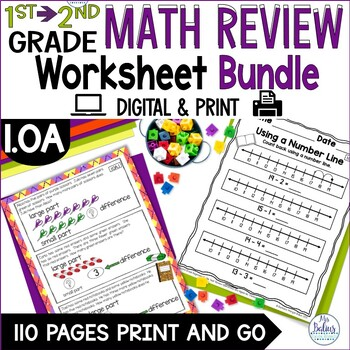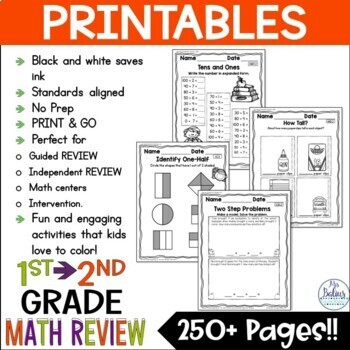DID YOU KNOW:
Seamlessly assign resources as digital activities

Learn how in 5 minutes with a tutorial resource. Try it Now

Learn More# Welcome to Second Grade! BOY Math Review #1 Common Core MathContent.OA1st - 3rd
Subjects
Standards
Resource Type
Formats Included
• PDF
Pages
55 pages

#### Also included in

1. Welcome to Second Grade! Second Grade Beginning of the Year Review BUNDLE. This is a bundle of my four beginning-of-the-year reviews that cover every first grade Common Core Math Standard.This unit is completely revised and edited!View the individual products below:Welcome to Second Grade! BOY Mat
\$16.00
\$32.00
Save \$16.00

### Description

Welcome to Second Grade!: Second Grade Beginning of Year Review #1 (Common Core 1.OA). This is the first of four beginning-of-year reviews that cover every first grade Common Core Math Standard. This resource reviews all of the standards under the OPERATIONS AND ALGEBRAIC THINKING strand of the first grade math standards. The review provides students practice of all of the skills required for mastery of the standards and allows a rigorous path that leads students into the next level of learning.

You may find the other reviews that are part of this series here:

Welcome to Second Grade! BOY Math Review #2 Common Core MathContent.NBT

Welcome to Second Grade! BOY Math Review #3 Common Core MathContent.MD

Welcome to Second Grade! BOY Math Review #4 Common Core MathContent.G

You may purchase all four at a reduced price here:

Welcome to Second Grade! BOY Math Review BUNDLE CCSS Aligned (All four reviews!)

The unit includes guided and independent practice for each concept. For each topic there is at least one colorful guided practice/re-teaching page designed for projection or for small group intervention. Also included are several printer-friendly (black and white and less graphics) pages for independent practice, to use for small group work or centers. Use part or all of this resource for your beginning-of-year review of the operations and algebraic thinking strand of the Common Core Math standards. (1.OA)

Standards included:

Operations and Algebraic Thinking 1.OA

Addition and Subtraction Word Problems (1.OA.1)

Two-Part Word Problems (1.OA.2)

Write the Addition Facts Another Way (1.OA.3)

Addition and Subtraction within Twenty (1.OA.5)

Balancing Equations (1.OA6)

Addition and Subtraction true or false (1.OA.7)

Find the missing number (1.OA.8)

I use ‘guided math’ in my classroom. I begin my teaching time block teaching/spiraling math concepts as a whole group, but most of my math block is devoted to guided practice in small groups followed by independent or small group practice, centers and intervention. Included in this resource are multiple guided practice pages for each topic that can be used for small group work or for intervention. I have also included lots of independent practice pages with less graphics for ease in printing.

Mrs. Balius

http://www.teacherspayteachers.com/Store/Mrs-Balius

http://pinterest.com/rsbalius/

http://mrsbalius.blogspot.com/

Check out my products in the Number of the Day Series:

Purchase the whole year here:

Number of the Day {The Whole Year!} Bundle

Number of the Day Units:

Number of the Day {Going Back to School} First Grade Math

Number of the Day {Fall Fun} First Grade Math

Number of the Day {Silly Scary Stuff!} First Grade Math

Number of the Day {Giving Thanks!} First Grade Math November

Number of the Day {Celebrate!} First Grade Math December

Number of the Day {Winter Wonderland} First Grade Math January

Number of the Day {Laissez le Bons Temps Rouler!} First Gr February

Number of the Day {Going to the Rodeo!} First Grade Math March

Number of the Day {Signs of Spring} First Grade Math April

Number of the Day {FIESTA!} First Grade Math May

Number of the Day {Going to the Beach} First Grade Math

Number of the Day {Going Camping!} First Grade Math July

Bundles:

Number of the Day {Back to School, Fall, October} Bundle

Number of the Day {November, December, January} Bundle

Number of the Day {Feb, March, April} FIrst Grade Math Bundle

Number of the Day {May, June, July} First Grade Math Bundle IV

Number of the Day {Going Back to School} Second Grade Math

Number of the Day {Winter Wonderland} Second Grade Math January

In Spanish:

Total Pages
55 pages
N/A
Teaching Duration
N/A
Report this Resource to TpT
Reported resources will be reviewed by our team. Report this resource to let us know if this resource violates TpT’s content guidelines.

### Standards

to see state-specific standards (only available in the US).
Partition circles and rectangles into two and four equal shares, describe the shares using the words halves, fourths, and quarters, and use the phrases half of, fourth of, and quarter of. Describe the whole as two of, or four of the shares. Understand for these examples that decomposing into more equal shares creates smaller shares.
Compose two-dimensional shapes (rectangles, squares, trapezoids, triangles, half-circles, and quarter-circles) or three-dimensional shapes (cubes, right rectangular prisms, right circular cones, and right circular cylinders) to create a composite shape, and compose new shapes from the composite shape.
Distinguish between defining attributes (e.g., triangles are closed and three-sided) versus non-defining attributes (e.g., color, orientation, overall size); build and draw shapes to possess defining attributes.
Determine the unknown whole number in an addition or subtraction equation relating three whole numbers. For example, determine the unknown number that makes the equation true in each of the equations 8 + ? = 11, 5 = ▯ - 3, 6 + 6 = ▯.
Understand the meaning of the equal sign, and determine if equations involving addition and subtraction are true or false. For example, which of the following equations are true and which are false? 6 = 6, 7 = 8 - 1, 5 + 2 = 2 + 5, 4 + 1 = 5 + 2.